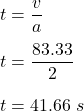79. If the takeoff velocity of an airplane on a runway is 300 km/hr with an acceleration of 2 m/s. What is the takeoff time of the air

Question

79. If the takeoff velocity of an airplane on a runway is 300 km/hr with an acceleration of 2 m/s. What is the takeoff time
of the airplane.

in progress 0
6 months 2021-07-26T02:23:08+00:00 1 Answers 16 views 0

The take off time is equal to 41.66s.

Explanation:

Given that,

The initial speed of the airplane, u = 0

Acceleration, a = 2 m/s²

Let v is the take off time. Using first equation of motion,

v = u +at

Put v = 300 km/hr = 83.3 m/s

So,So, the take off time is equal to 41.66s.